## Digital Control of Power Stage Voltage

This example shows how to tune a high-performance digital controller with bandwidth close to the sampling frequency.

### Voltage Regulation in Power Stage

We use Simulink to model the voltage controller in the power stage for an electronic device:

```open_system('rct_powerstage') ```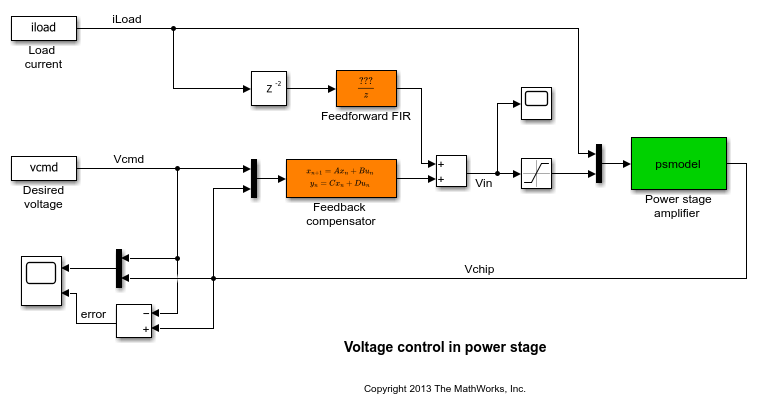The power stage amplifier is modeled as a second-order linear system with the following frequency response:

```bode(psmodel) grid ```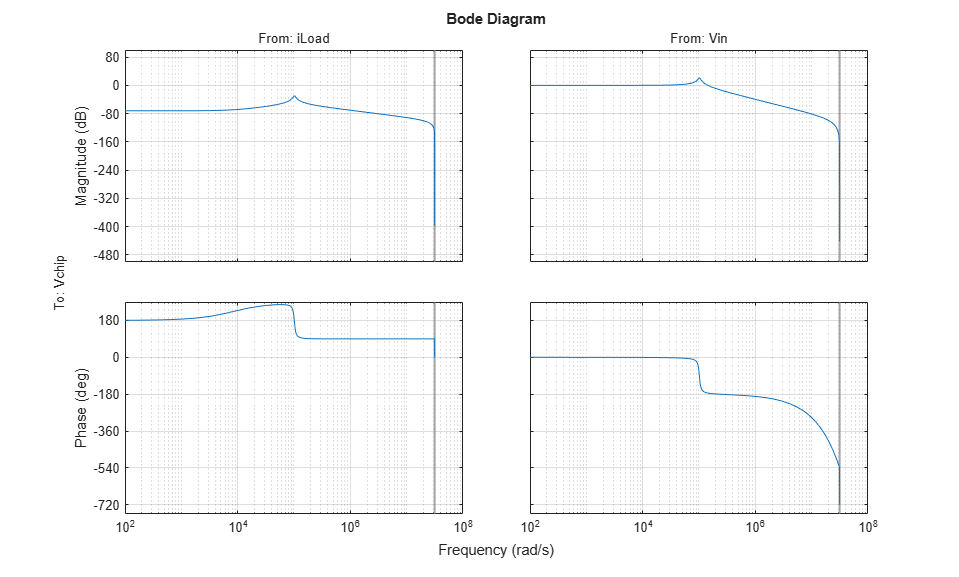The controller must regulate the voltage `Vchip` delivered to the device to track the setpoint `Vcmd` and be insensitive to variations in load current `iLoad`. The control structure consists of a feedback compensator and a disturbance feedforward compensator. The voltage `Vin` going into the amplifier is limited to. The controller sampling rate is 10 MHz (sample time `Tm` is 1e-7 seconds).

### Performance Requirements

This application is challenging because the controller bandwidth must approach the Nyquist frequency `pi/Tm` = 31.4 MHz. To avoid aliasing troubles when discretizing continuous-time controllers, it is preferable to tune the controller directly in discrete time.

The power stage should respond to a setpoint change in desired voltage `Vcmd` in about 5 sampling periods with a peak error (across frequency) of 50%. Use a tracking requirement to capture this objective.

```Req1 = TuningGoal.Tracking('Vcmd','Vchip',5*Tm,0,1.5); Req1.Name = 'Setpoint change'; viewGoal(Req1) ```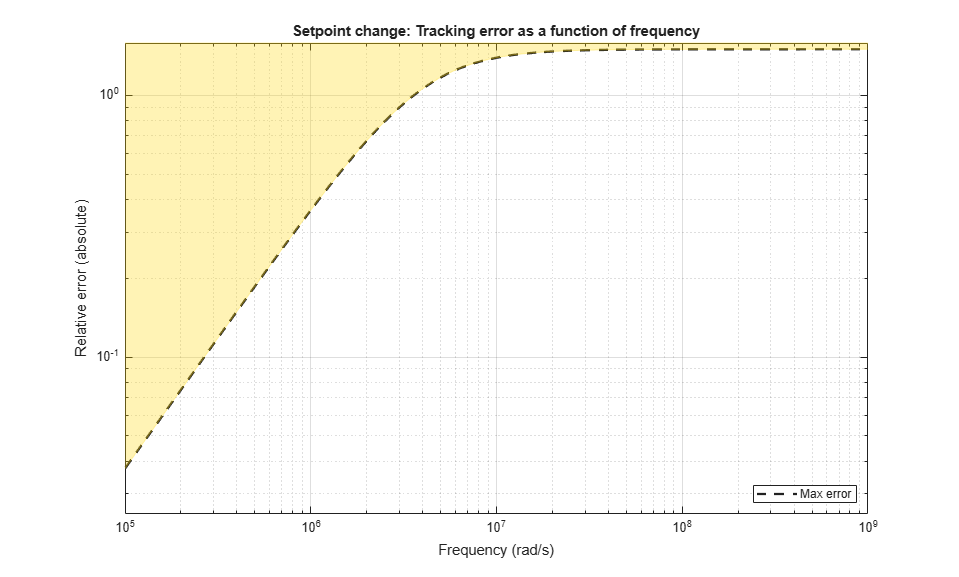The power stage should also quickly reject load disturbances `iLoad`. Express this requirement in terms of gain from `iLoad` to `Vchip`. This gain should be low at low frequency for good disturbance rejection.

```s = tf('s'); nf = pi/Tm; % Nyquist frequency Req2 = TuningGoal.Gain('iLoad','Vchip',1.5e-3 * s/nf); Req2.Focus = [nf/1e4, nf]; Req2.Name = 'Load disturbance'; ```

High-performance demands may lead to high control effort and saturation. For the ramp profile `vcmd` specified in the Simulink model (from 0 to 1 in about 250 sampling periods), we want to avoid hitting the saturation constraint. Use a rate-limiting filter to model the ramp command, and require that the gain from the rate-limiter input tobe less than.

```RateLimiter = 1/(250*Tm*s); % models ramp command in Simulink % |RateLimiter * (Vcmd->Vin)| < Vmax Req3 = TuningGoal.Gain('Vcmd','Vin',Vmax/RateLimiter); Req3.Focus = [nf/1000, nf]; Req3.Name = 'Saturation'; ```

To ensure adequate robustness, require at least 7 dB gain margin and 45 degrees phase margin at the plant input.

```Req4 = TuningGoal.Margins('Vin',7,45); Req4.Name = 'Margins'; ```

Finally, the feedback compensator has a tendency to cancel the plant resonance by notching it out. Such plant inversion may lead to poor results when the resonant frequency is not exactly known or subject to variations. To prevent this, impose a minimum closed-loop damping of 0.5 to actively damp of the plant's resonant mode.

```Req5 = TuningGoal.Poles(0,0.5,3*nf); Req5.Name = 'Damping'; ```

### Tuning

Next use `systune` to tune the controller parameters subject to the requirements defined above. First use the `slTuner` interface to configure the Simulink model for tuning. In particular, specify that there are two tunable blocks and that the model should be linearized and tuned at the sample time `Tm`.

```TunedBlocks = {'compensator','FIR'}; ST0 = slTuner('rct_powerstage',TunedBlocks); ST0.Ts = Tm; % Register points of interest for open- and closed-loop analysis addPoint(ST0,{'Vcmd','iLoad','Vchip','Vin'}); ```

We want to use an FIR filter as feedforward compensator. To do this, create a parameterization of a first-order FIR filter and assign it to the "Feedforward FIR" block in Simulink.

```FIR = tunableTF('FIR',1,1,Tm); % Fix denominator to z^n FIR.Denominator.Value = [1 0]; FIR.Denominator.Free = false; setBlockParam(ST0,'FIR',FIR); ```

Note that `slTuner` automatically parameterizes the feedback compensator as a third-order state-space model (the order specified in the Simulink block). Next tune the feedforward and feedback compensators with `systune`. Treat the damping and margin requirements as hard constraints and try to best meet the remaining requirements.

```rng(0) topt = systuneOptions('RandomStart',6); ST = systune(ST0,[Req1 Req2 Req3],[Req4 Req5],topt); ```
```Final: Soft = 1.29, Hard = 0.99482, Iterations = 545 Final: Soft = 1.28, Hard = 0.99791, Iterations = 501 Final: Soft = 1.29, Hard = 0.99553, Iterations = 543 Final: Soft = 1.29, Hard = 0.99057, Iterations = 419 Final: Soft = 1.29, Hard = 0.95154, Iterations = 435 Final: Soft = 1.28, Hard = 0.9997, Iterations = 507 Final: Soft = 1.29, Hard = 0.97804, Iterations = 398 ```

The best design satisfies the hard constraints (`Hard` less than 1) and nearly satisfies the other constraints (`Soft` close to 1). Verify this graphically by plotting the tuned responses for each requirement.

```figure('Position',[10,10,1071,714]) viewGoal([Req1 Req2 Req3 Req4 Req5],ST) ```### Validation

First validate the design in the linear domain using the `slTuner` interface. Plot the closed-loop response to a step command `Vcmd` and a step disturbance `iLoad`.

```figure('Position',[100,100,560,500]) subplot(2,1,1) step(getIOTransfer(ST,'Vcmd','Vchip'),20*Tm) title('Response to step command in voltage') subplot(2,1,2) step(getIOTransfer(ST,'iLoad','Vchip'),20*Tm) title('Rejection of step disturbance in load current') ```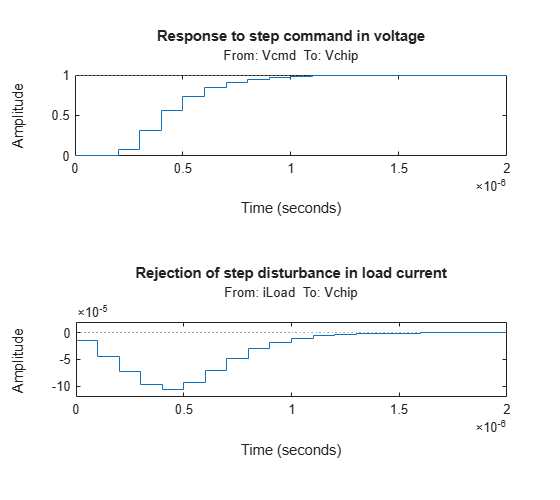Use `getLoopTransfer` to compute the open-loop response at the plant input and superimpose the plant and feedback compensator responses.

```clf L = getLoopTransfer(ST,'Vin',-1); C = getBlockValue(ST,'compensator'); bodeplot(L,psmodel(2),C(2),{1e-3/Tm pi/Tm}) grid legend('Open-loop response','Plant','Compensator') ```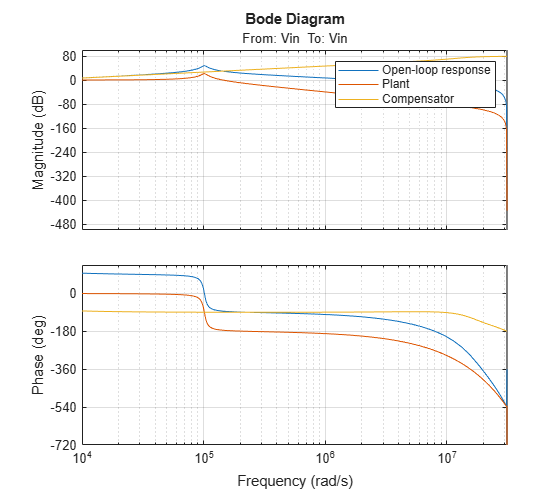The controller achieves the desired bandwidth and the responses are fast enough. Apply the tuned parameter values to the Simulink model and simulate the tuned responses.

```writeBlockValue(ST) ```

The results from the nonlinear simulation appear below. Note that the control signal `Vin` remains approximately withinsaturation bounds for the setpoint tracking portion of the simulation.Figure 1: Response to ramp command and step load disturbances.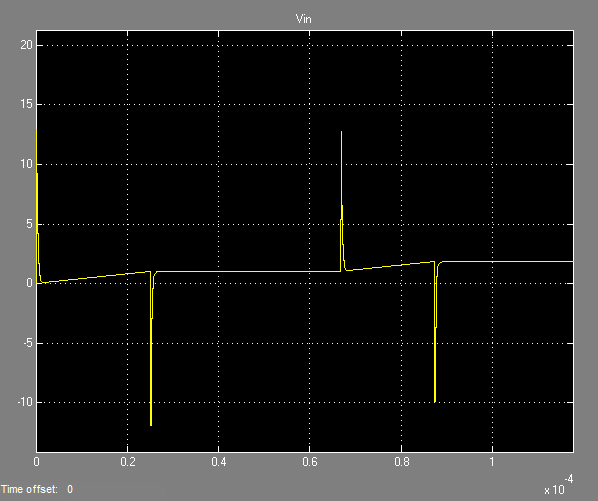Figure 2: Amplitude of input voltage `Vin` during setpoint tracking phase.